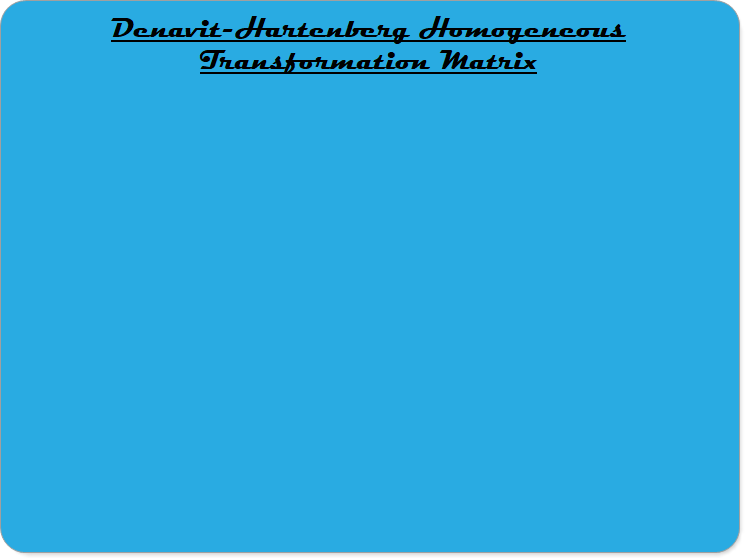Robotics 2
Denavit-Hartenberg Forward Kinematics
In this video, you are introduced to a method called 'Denavit-Hartenberg' for finding the Homogeneous Transformation Matrix for a robot serial manipulator.In this video, you are given the four rules for assigning frames according to the Denavit-Hartenberg method for forward kinematics.In this video, you are given the definitions of the four Denavit-Hartenberg parameters, and one complete example of finding the parameters for a 3-degree-of-freedom manipulator.
To complete this lab activity, do the following:
(1) Draw a kinematic diagram for your SCARA manipulator
(2) Find the homogeneous transformation matrix for your SCARA manipulator (which you built in the last section) using the Denavit-Hartenberg method
(3) Plug in some values for Theta 1, Theta 2, and d3 and calculate the position of the end-effector at those values

Make a video that shows: